-
| /
Save up to %
Save %
Save up to
Save
Sale
Sold out
In stock
Search

# How to Calculate Electric Motor Torque 2023

Published: 21-06-2022, Last updated 21-06-2022

## Introduction

Torque is an important variable when considering electric motor performance. It can be used to calculate mechanical power and derive electrical power. For drones and electric aircraft, knowing your torque also allows you to calculate motor efficiency separately from the efficiency of the rest of the system.

• Use the electric motor torque formula to calculate the torque of a brushless motor
• Test our calculation experimentally using the Series 1585 thrust stand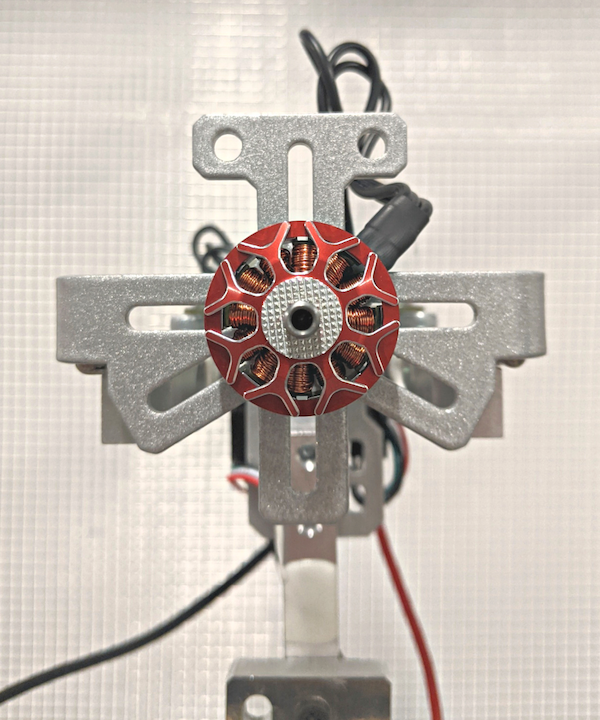Figure 1: Series 1585 thrust stand with brushless motor

## So how can you calculate electric motor torque?

Let’s say we want to know the torque of our motor, the Xoar 2407. Here’s what we know:

• Weight: 36 g
• Kv: 2300 Kv
• Stator dimensions: 24 x 7 x 0.15 mm
• Config: 12N14P

Nothing here is immediately helpful for calculating torque, so let’s look at some background information.

Motor torque is related to several variables, but the most important one for calculating torque is current.

### Electric Motor Torque Formula:‘Kt’ is the motor torque coefficient, whose units are N.m/A (Newton-meters per Amp). Kt is the ratio of torque:current, a relationship that is not perfectly linear.

By itself, this coefficient is not of much use to us, but we can use the assumption that Kt = 1/Kv, and Kv is a number that we do have.

Let’s say we want to deliver 20 A of current to our motor. We can use this number and our motor’s Kv to calculate the theoretical torque.

Motor Kv is generally given in RPM/V, but for it to work in our formula we need it in SI units, which is (Radians/ Second)/ Volt in this case. To make the conversion we will divide 2300 by 60 s and multiply it by 2π.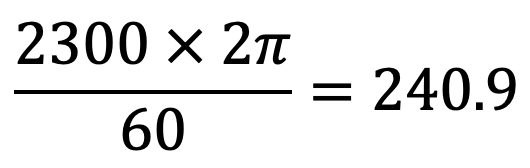For a more precise explanation of motor Kv see our article: How to Calculate Motor Kv

Now we can plug our SI Kv value into our motor torque formula: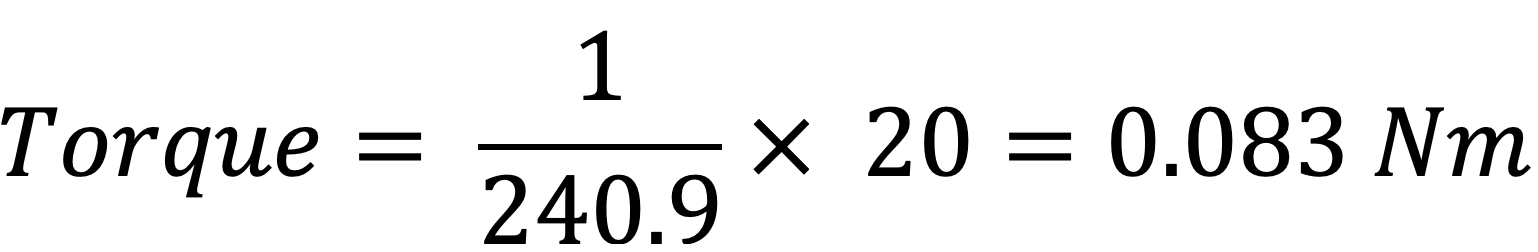This figure seems reasonable, but the nonlinearity caused by the ESC and the motor can mean that theoretical results do not necessarily reflect reality. We generally expect a difference of 10 - 50% between the theoretical value and the measured value.

Note that this equation works on the assumption that the relationship between torque and current is linear, which is not the case, so the torque calculated will not be perfectly accurate.

Let’s hook up the motor to our test stand and see how close we get to our theoretical value.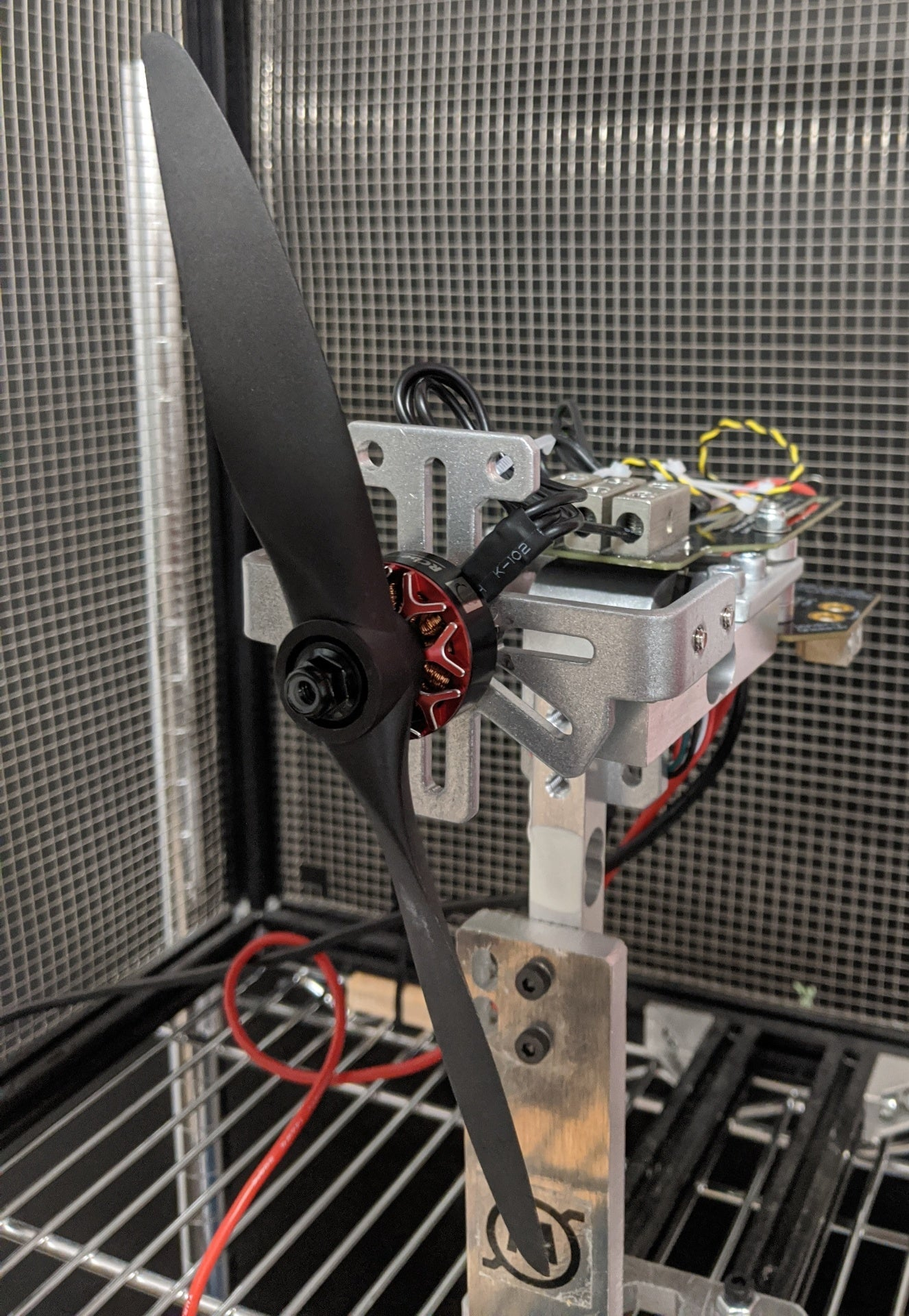Figure 2: Series 1585 test stand with brushless motor and propeller

We used the test stand to run a simple step test, taking a measurement when the system reached 20 A. The results of that test are below.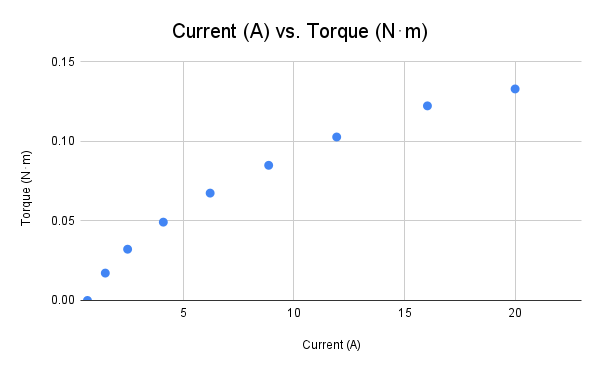Figure 3: Torque vs. Current results

At 20 A, we measured 0.133 Nm of torque with the Series 1585, a 46% difference from what we calculated.

As you can see, contrary to what the formula suggests, the relationship between current and torque is not linear. This is due to losses from the ESC and the motor.

This data goes to show that torque calculations can only give us an estimate of the true torque produced by a motor. To obtain accurate torque data, you need a tool to measure it.

We’ve been aware of this dilemma for quite some time, which is why we developed our motor test stands to allow users to collect highly accurate motor data:

Further reading: Brushless Motor Power and Efficiency Analysis

If you have any further questions don’t hesitate to leave us a comment below.

### Chris

August 29, 2023

Could you elaborate how you measure the torque based on the two load cells? I have a hard time understanding that. Optimally each of the load cells measure the same load (just from different direction) while the motor with the prop is spinning. Additionally we know the spacing between the load cells, or the center of the spinning shaft and one load cell. But which formula are those values then plugged into to arrive at the torque?

### Charles Blouin - Tyto Robotics staff

May 25, 2023

@Tushar The mechanical power is going to be exactly torque * rotation speed. With SI units, you get Watts directly. In practice, torque should be measured experimentally because, as seen in the article above, the linear model causes a significant error.

### Tushar

May 23, 2023

Hi
So, Is there no formula which can calculate mechanical power theoretically for an bldc motor , waiting for you response.

### SAMWEL

February 21, 2023

Can I get electrical reading materials, ( Notes) on pdf from you??

### Lauren Nagel

February 20, 2023

Hi Ed,

Thanks for your comment. This is in fact a Xoar 2407 2300Kv motor, it is a custom motor that Xoar provided us for our Series 1580 Education kits. Here’s a photo for reference: https://cdn.tytorobotics.com/blog_posts/xoar-2407-2300kv-motor.JPG

- Lauren

### ed

February 20, 2023

HI,
I don think you used a Xoar 2407 2300kv here, i think you used a 1500kv variant, this would match with you maths, i dont think the 2300kv exists